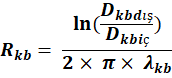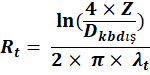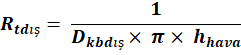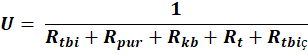﻿ Calculation of Heat Loss-Pipe Thermal Conductivity Resistancex
Calculation of Pipe Diameter

In our day, energy and the efficient use of energy are among the most important factors, and the main purpose of use of pre-insulated pipes is to keep heat loss at the lowest possible level and obtain the highest efficiency from energy. The calculation method given below can be used for calculating the heat loss theoretically.

First of all, the average fluid temperature is calculated:Tm (˚C) : Average temperature of the fluid

Tg (˚C) : Temperature at the direction of flow

Td (˚C) : Temperature at the direction of return

Tt (˚C) : Outdoor air temperature

In order to calculate the total thermal conductivity resistance of the pre-insulated pipe system, firstly the resistances of each layer of the system must be calculated respectively.

Carrier Pipe Thermal Conductivity Resistance:Rtbi (m.°C / W) : Thermal conductivity resistance of the carrier pipe

Din (m) : Inner diameter of the carrier pipe

Dout (m) : Outer diameter of the carrier pipe

λtb(W / m.°C) : Carrier pipe thermal conductivity coefficient (Table 1)

 Type of the Service Pipe Thermal Conductivity Coefficient (W/m.˚C) Black Steel 76 Stainless Steel 16 PP-R 0,15 Copper 400 CTP 0,31 Polyethylene 0,43

Table 1: Thermal Conductivity Coefficient by Types of Carrier Pipe

Polyurethane Insulation Thermal Conductivity Resistance:

Rpur= ln(DkbinDout)2× π× λpurRpur(m.°C / W) : PUR insulation thermal conductivity resistance

Dkbin(m): Inner diameter of the casing pipe

λpur(W / m.°C): PUR insulation thermal conductivity coefficient (0.026 W / m.°C can be taken)

Thermal Conductivity Resistance of the HDPE Casing Pipe:Rkb(m.°C / W) : Thermal conductivity resistance of the casing pipe

Dkbout(m): Outer diameter of the casing pipe

Dkbin(m): Outer diameter of the casing pipe

λkb(W / m.°C): Thermal conductivity resistance of the casing pipe

Outdoor Thermal Conductivity Resistance:

• Underground InstallationRt(m.°C / W) : Thermal conductivity resistance of earth

Z(m) : Filling height of earth

λt(W / m.°C): Thermal conductivity coefficient of earth (Table 2)

 Type Density (kg/m3) Volumetric Humidity Rate % Thermal Conductivity Coefficient (W/m.˚C) Sand 1500 4 1,04 1800 14 1,7 Clay Soil 1500 23 1,5 2000 28 2,6

Table 2: Thermal Conductivity Coefficient of Earth

• Ground InstallationRtdout(m.°C / W): Outer convection resistance of the casing pipe

hair(W / m².°C): Air convection coefficient

Thermal Conductivity Resistance between Flow - Return PipesRtbin(m.°C / W): Thermal conductivity resistance between flow - return pipes

C (m) : Distance between the axes of flow - return pipes

After calculating all resistance factors, the total thermal conductivity resistance value of the pre-insulated pipe system can be calculated through the formulas below:

• If under the ground:• If above the ground:U (W/m.˚C) : Total thermal conductivity coefficient

Total heat loss per metre in pre-insulated pipes:

Q=U× ToQ (W / m) : Total heat loss per metre

The formula below can be used to calculate the fluid temperature at the end of the line according to all calculations:L (m) : Length of line

m (kg/sec) : Water flowrate

Cp(J / kg.°C) : Fluid specific heat [Table 3]

Tson(°C) : Fluid final temperature

 Temperature (˚C) Density (kg/m3) Specific Heat (Kj/kg ˚C) 0 1000 4210 5 1000 4204 10 1000 4193 20 998 4183 30 996,02 4179 40 992,06 4179 50 988,14 4182 60 983,28 4185 70 977,52 4191 80 971,82 4198 90 965,25 4208 100 957,85 4219

Table 3: Physical Specifications of Water

;